# Axiomatized class

A class of models of one type, defined by an axiom system. A classof models of a formal language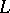is said to be axiomatized (finitely axiomatized) if there exists a (finite) system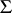of closed formulas ofsuch thatcontains those and only those models on which all formulas ofare defined and are true (cf. Algebraic system). A class of models of a recursive signature is said to be recursively axiomatized if it can be specified by a recursive set of axioms.

Many classes of algebraic systems studied in mathematics are defined by a system of axioms of a first-order language. For instance, the classes of all Boolean algebras, all groups, all fields, and all lattices are finitely axiomatized. The classes of all torsion-free groups, all fields of characteristic zero and all algebraically closed fields are recursively axiomatized, but not necessarily finitely axiomatized. The theory of axiomatized classes reveals regularities common to all classes of objects defined by a specific language; it has been well developed for first-order languages, and therefore only such classes and formulas will be dealt with in what follows.

Two models are said to be elementarily equivalent if any formula of the first-order language which is true in one of them is also true in the other. A model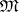is said to be an elementary extension of a modelif any formula which is defined and is true inis also true in.

An elementary closed classof models is called complete if all its models are elementarily equivalent. Every axiomatized class of models is a sum of pairwise-disjoint complete classes. A class is said to be categorical in cardinalityif all its models of cardinality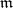are isomorphic. A complete class of models of a countable signature that is categorical in an uncountable cardinality, is categorical in all uncountable cardinalities, but may be non-categorical in a countable cardinality; in such a case the class has a countable number of pairwise non-isomorphic countable models. For any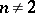there exists a complete axiomatized class with exactlynon-isomorphic countable models.

An axiomatized classof models is called solvable if there exists an algorithm by which it is possible to tell, for any closed formula of the language, if it is true or false for each model in. The following theorem describes the interconnection of complete, categorical and solvable classes: Ifis categorical of infinite cardinality and has no finite model, then it is complete. A recursively-complete axiomatized class of models is solvable.

Reductive and projective classes are generalizations of axiomatized clases. Projective classes are defined by a second-order axiom in the formwhere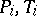are predicate variables, and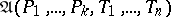is a formula of the signature. Many properties of axiomatized classes can be applied to these classes.